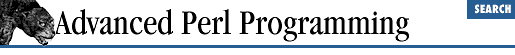#Chapter 5Eval## 5.4 Using Eval for Expression Evaluation

There are a number of tasks, such as parsing and expression evaluation, in which you can make Perl do all the dirty work. Assuming, of course, that your parsing requirements are similar to Perl's own. Perl, after all, knows a thing or two about parsing and evaluating Perlish statements!

Let's assume that your input data is a bunch of quoted strings and you would like to verify that the quotes are balanced:

```'He said, "come on over"'
'There are times when "Peter" doesn\'t work at all'```

Instead of fretting over backslash escapes and writing code to check whether the quotes are correctly paired (balanced), you can simply eval the strings, as shown in Example 5.1. Remember that a string is a correct Perl expression too. If Perl puts an error in \$@, you can be sure you have faulty input.

#### Example 5.1: eval.pl

```while (defined(\$s = <>)) {          # Read a line into \$s
\$result = eval \$s;              # Evaluate that line
if (\$@) {                       # Check for compile or run-time errors.
print "Invalid string:\n \$s";
} else {
print \$result, "\n";
}
}```

The neat thing about this code is that it works equally well as a fancy calculator, because \$s can be any valid Perl statement, with arithmetic operators, loops, variable assignments, subroutines, and so on. Here's how you might use the program:

```% perl eval.pl
2 * log (10);
4.60517018598809
\$a = 10; \$a += \$a ** 2;
110
for (1..10) {print \$_ , " " }
1 2 3 4 5 6 7 8 9 10```

For each line you enter, Perl computes and prints out the result (shown in non-bold type). How much simpler a shell can you possibly ask for? Note that the code requires each input line to be a fully formed expression, so you cannot write multiline expressions, but you can always change the program to start evaluating only when the user enters a blank line.

It is worth contrasting these few lines with the effort of learning lex and yacc - see, for example, the yacc, lex, and C-based calculator ("hoc") developed by Kernighan and Pike in their classic, The Unix Programming Environment. Other static languages such as Java and C++ present similar challenges: you are forced to reinvent the wheel, since you don't have access to the power of the compiler itself.

### 5.4.1 Expression Evaluation in Substitutions

The Perl substitution operator is ordinarily of the form s/`regex`/`replacement`/ and substitutes the replacement string wherever the input string matches the regular expression pattern. The /e flag adds a twist to this: it tells the substitution operator that the second part is a Perl expression, not an ordinary replacement string; the result of the expression is used as the replacement instead. Consider

```\$line = 'Expression Evaluation';
\$line =~ s/(\w+)/ scalar (reverse(\$1)) /eg;
print \$line; # prints "noisserpxE noitaulavE"```

The second parameter to the substitute operator is an expression: reverse is used in a scalar context to reverse the string given to it. The /g flag ensures that every word is matched and reversed.

This topic is somewhat tangential to the eval keyword, but it is still germane to our discussion about run-time expression evaluation; in fact, /e stands for "expression," not for "eval." This expression is checked for syntax at compile-time, so if you need to watch for run-time errors, you still need to put the entire statement within an eval block. Consider another example, which replaces any string containing the pattern "number/number" with an equivalent fraction:

```\$l = 'His chances of winning are between 2/5 and 1/3';
eval {
\$l =~ s|(\d+)/(\d+)| \$1 / \$2 |eg;
};
print \$l unless \$@;```

This prints "His chances of winning are between 0.4 and 0.333333333333333." The eval block traps divide-by-zero errors.